13. NUCLEI

Q.1. Define binding energy of a nucleus.

Ans ⇒ It is defined as the amount of work done to separate the nucleons an infinite distance apart from each other so that they no longer interact with each other.
Or. It is defined as the energy equivalent to the mass defect of the nucleus. Mathematically,
B.E. = Δmc2 (J) = Δm x 931.5 MeV.
Where Δm = mass defect of nucleus, c = 3 x 108 ms-1 = speed of light.

Q.2. Define the following:
(a) Mass defect
(b) Binding energy per nucleon
(c) Packing fraction of a nucleus.

Ans ⇒ (a) Mass defect : It is defined as the difference between the sum of the masses of nucleons constituting a nucleus and the rest mass of the nucleus.

(b) Binding energy per nucleon : It is defined as the average energy required to extract one nucleon from the nucleus.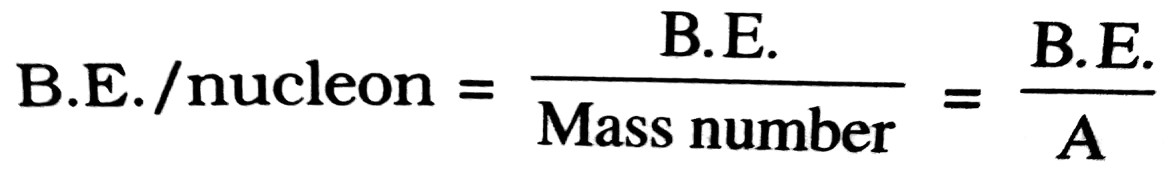(c) Packing fraction of a nucleus : It is defined as the mass defect per nucleon of the nucleus.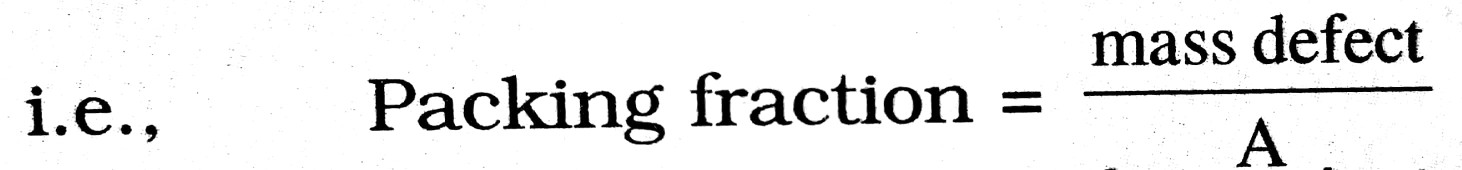Q.3. What holds the nucleons together in a nucleus ?

Ans ⇒ The nucleons are held inside the nucleus due to the nuclear force between them which is basically a strong attractive force although the electrostatic force of repulsion between the protons is very large inside the nucleus.

Q.4. How the stability of a nucleus is decided ?

Ans ⇒ The stability of a nucleus is decided by its binding energy per nucleon and not by its binding energy. Higher the value of B.E. per nucleon of a nucleus greater will be its stability and vice-versa.

Ans ⇒ It is defined as the substance whose atoms have unstable nuclei and emit ∝, β and γ-rays.

Q.6. Define Curie.

Ans ⇒ It is defined as the quantity of any radioactive substance which undergoes 3.7 x 1010 disintegrations per second. It is the S.I. unit of measuring the radioactivity of any radioactive substance.

Q.7. Define nuclear reactor. Name its main components.

Ans ⇒ Nuclear reactor : It is defined as a device in which nuclear chain reaction takes place in a controlled way.
Its main components are :
(a) Nuclear fuel
(b) Moderator
(c) Control rods or Cadmium rods
(d) Coolant
(e) Protective shield.

Q.8. What is the relationship between Half life and Decay constant ? Define mass defect.

Ans ⇒ From relation N = N0e-λt (From law of radioactivity)
we get,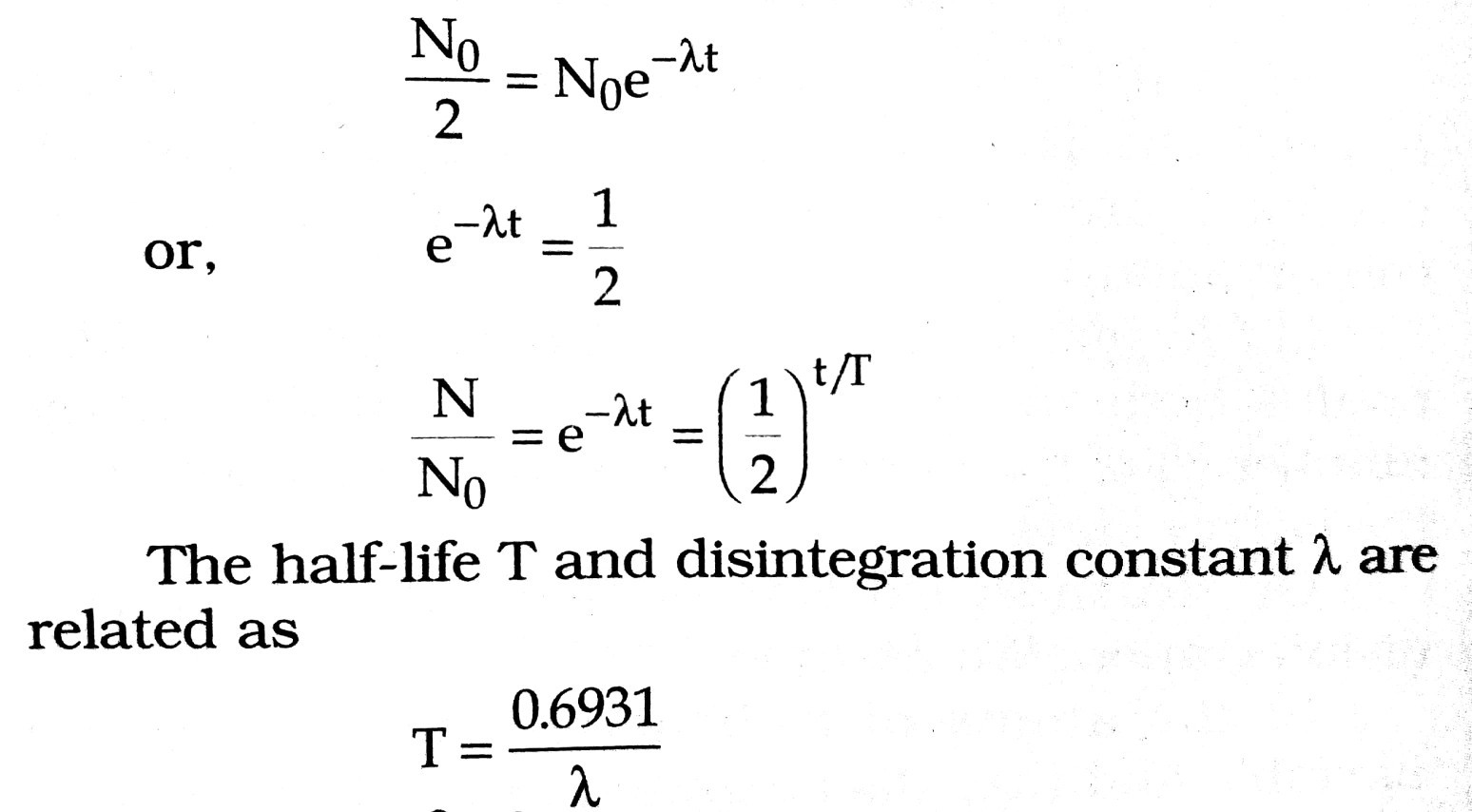Mass Defect : It is observed that the mass of a nucleus is always less then the mass of constituent nucleon (i.e., protons + neutrons). This difference of mass is called the mass defect. Let (Z, A) be the mass of nucleus, mp = the mass of proton and mn = mass of neutron, then the mass defect
Δm = Mass of nucleons – Mass of nucleus
= Zmp + (A – Z) mn – Mnucleus

Q.9. Explain the term distance of closest approach and deduce the expression for it.

Ans ⇒ Distance of closest Approach : The minimum distance upto which on energetic particle travelling directly towards the nucleus can move before coming to rest and retraces it path is called distance of closest approach.
Consider on particle of mass m moving directly towards a nucleus with velocity v at any given time. As the particle approaches nucleus at the distance of closest approach (r0) its K.E. is transferred to electrostatic P.E.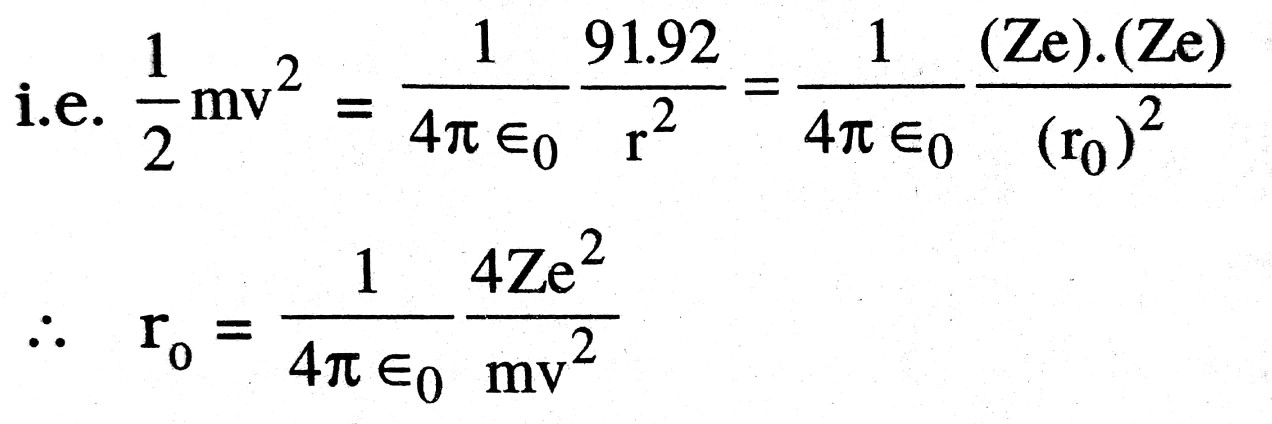Q.10. Give an account of the laws of radio activity.

Ans ⇒ Wheather the radioactivity is natural or artificial, it obeys the following laws :

(i) Soddy-Fajan’s displacement law : When a nucleus (X) emits an ∝-particle, the product (daughter) nucleus (Y) is displaced on the periodic table down by mass number 4 and by atomic number (charge number) 2.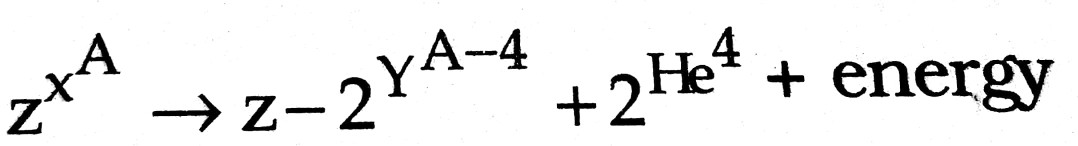(ii) β-(electron) or β+ (positron) : The product nucleus gets displaced up or down respectively by atomic number one without any displacement on the mass scale.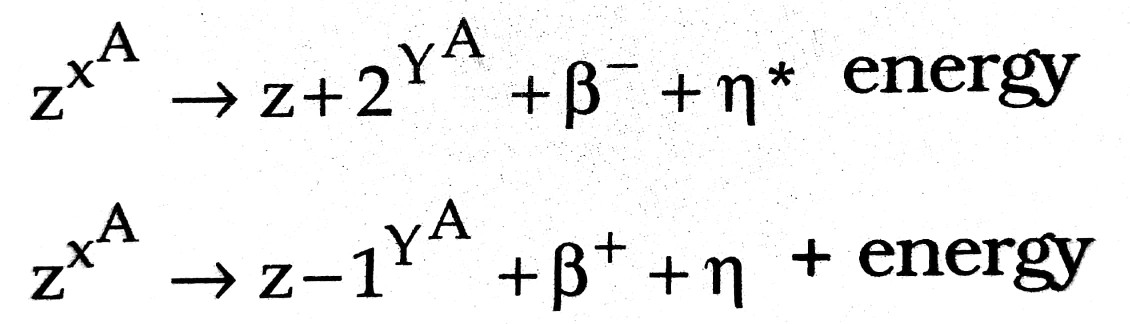η* and η are antineutrino and neutrino respectively. These are of zero charge and zero rest mass and are introduced to ensure the momentum conservation.

(iii) A γ(gamma) photon : The daughter nucleus retains both the mass number (A) and the charge number (2) of the parent nucleus but gets lowered in the energy state.

Or, Rutherford-Soddy laws of radioactive disintegration. We assume that :

(i) the atoms of radioactive substances are unstable and they disintegrated at random, that is, which particular atom of the substance disintegrates first depends upon the chance only, and

(ii) also as a very large number of radioactive atoms are present in a radioactive sample, so statistical method can be applied.
Suppose that at the time t there are N radioactive atoms. Let a number of atoms dN disintegrate in time dt. As the rate of disintegration is directly proportional to the number of atoms present.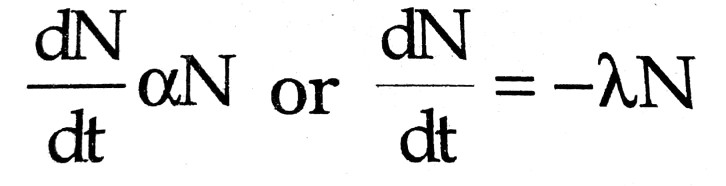λ is the radioactive constant and it depends upon the nature of the radioactive substance. The negative sign shows that with the increase in disintegration the value of N decreases.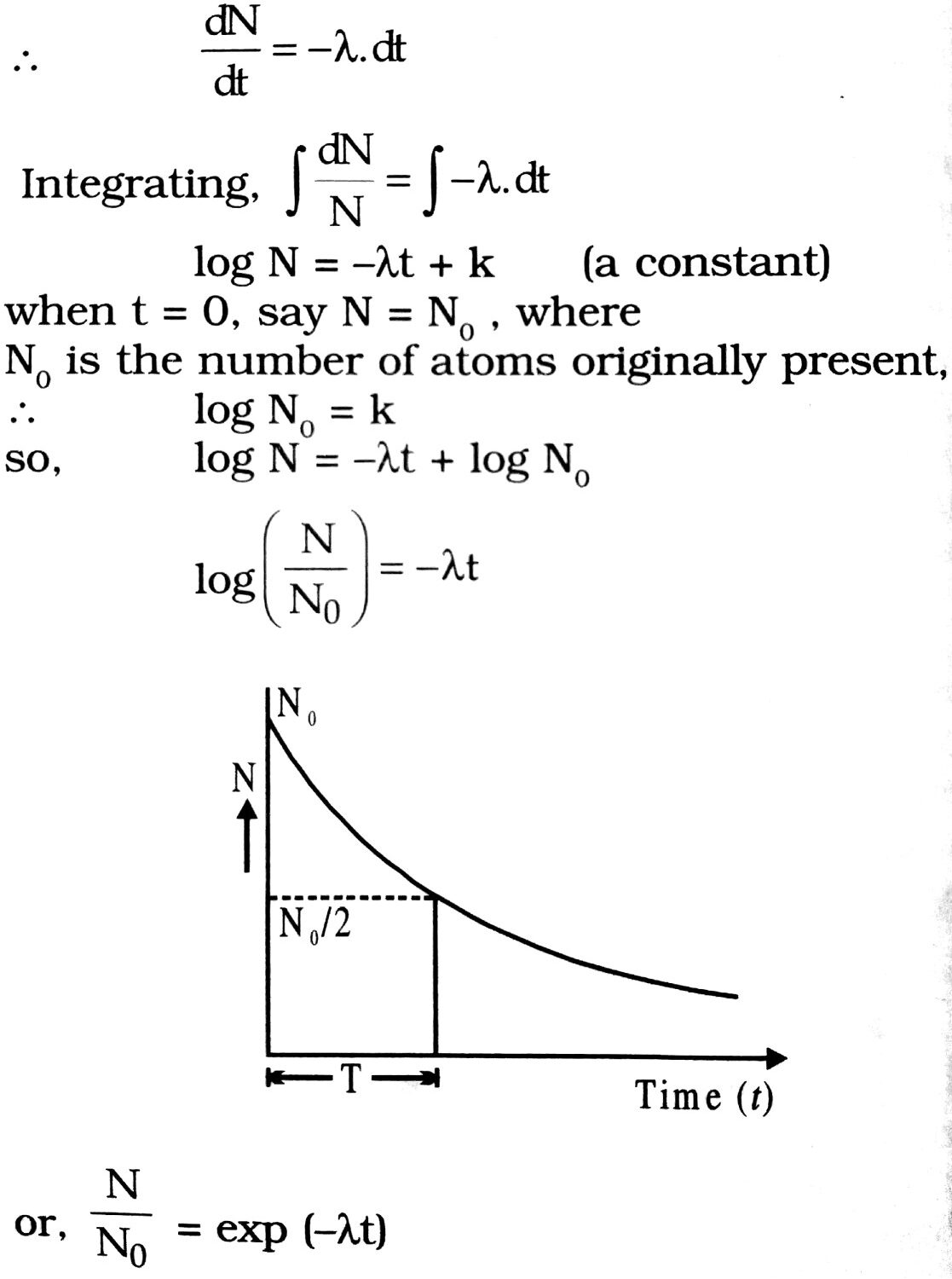∴   N = N0e-λt

Thus, the number of radioactive atoms decreases exponentially with time as shown in Fig.

Q.11. Define the terms half-life period and decay constant of a radioactive substance. Write their S.I. units. Establish the relationship between the two.

Ans ⇒ Half life (T) : Half-life period is the duration of time during which number of atoms left undecayed in the sample is half the total number of atoms present initially in the sample.
S. I. unit is second.

Decay constant (λ) : Decay constant of a radioactive element is the reciprocal of the time at the end of which the number of atoms left undecayed reduces to 37% of its initial value. S.I. unit is sec-1.
Relation between λ and T. We know N = N0 e-λT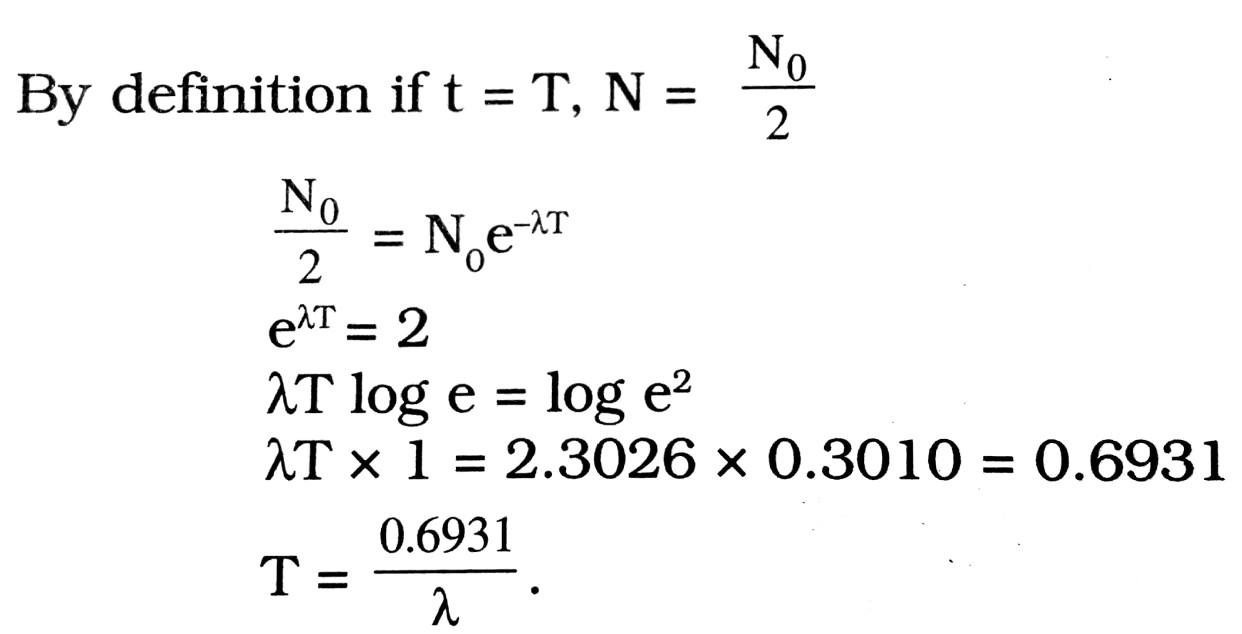Q.12. The activity of a source undergoing single type of decay is I, at time t = 0. Obtain an expression in terms half life T1/2 for the activity 1 at any subsequent time.

Ans ⇒ Applying law radioactive decay.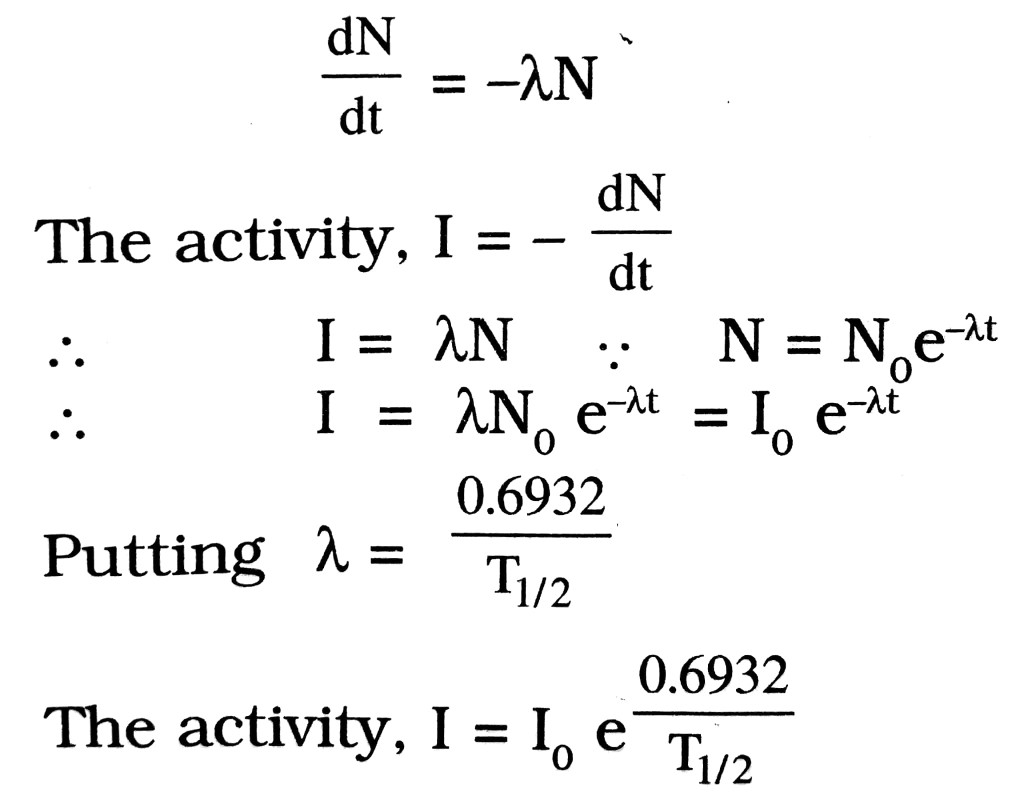where I0 is the activity at time t = 0

Q.13. What do you mean by nuclear fusion and nuclear fission ?

Ans ⇒ When two light nucleus fuse at very high temperature, comparatively heavier nucleus is formed. This process is called nuclear fusion. When four protons (Hydrogen nucleus) fuse together to form a helium nucleus, nearly 26 MeV energy is liberated. The mass defect is converted in form of energy. This process is possible at nearly 106 to 107 °C. The temp. of this order is obtained by nuclear explosion. Nuclear fusion is the main source of solar energy. Hydrogen bomb is based on this process.
Such nuclear phenomenon in which large amount of energy is released when a heavy nucleus breaks into two comparable nucleus, called nuclear fission.
92U235 + —-→ 0n1 —-→ 56Kr89 + 30n1 + Energy                                                                                    In this process also there is mass defect which is converted into energy. Atom bomb and nuclear reactor are based on this process.

Q.14. What do you mean by critical mass ?

Ans ⇒ Neutrons coming out after fission 92U23 are slowen down after travelling at least 10 cm so that they may produce fission again. If thickness of system is less than 10 cm then neutrons come out without doing their job. So, if size of matter is large then this action is possible. So for sustained fission the mass of matter should be greater than a certain mass. This minimum mass of material for performing fission is called critical mass.

Q.15. What is carbon dating ?

Ans ⇒ Carbon (C14) is produced in plants by the process of photosynthesis CO2 containing C14 is obtained by the action of high energy neutrons is cosmic rays on nitrogen and absorption of O2 by C14.
7N14 + 0n1 ——→ 6C14 + 1H1
All live plants and bones of animals have C14 which is in equilibrium with C14 in the atmosphere and remains constant so long as the plant or animal is alive, but after the same dies, radiocarbon C14 continues to disintegrate and number of disintegration per minute decreases with time. Thus the time by C14 to decay when the plant or animal died and ceased to assimilate C14, can be easily estimated.
The half life of C14 is 5730 years and it emits a β- particle of 0.155 MeV transforming itself back into N14. The age is calculated by this formula, N = N0e-λt and the value of N0 is 153 disintegration per minute per gram of carbon.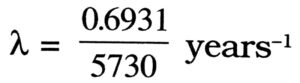After the death of the sample, the number of disintegration in any sample decreases substituting the number of disintegration N in any old sample, its age is calculated from the noted equation.

Q.16. How size of nucleus is related with mass number ?

Ans ⇒ The volume of nucleus is directly proportional to mass number. i.e.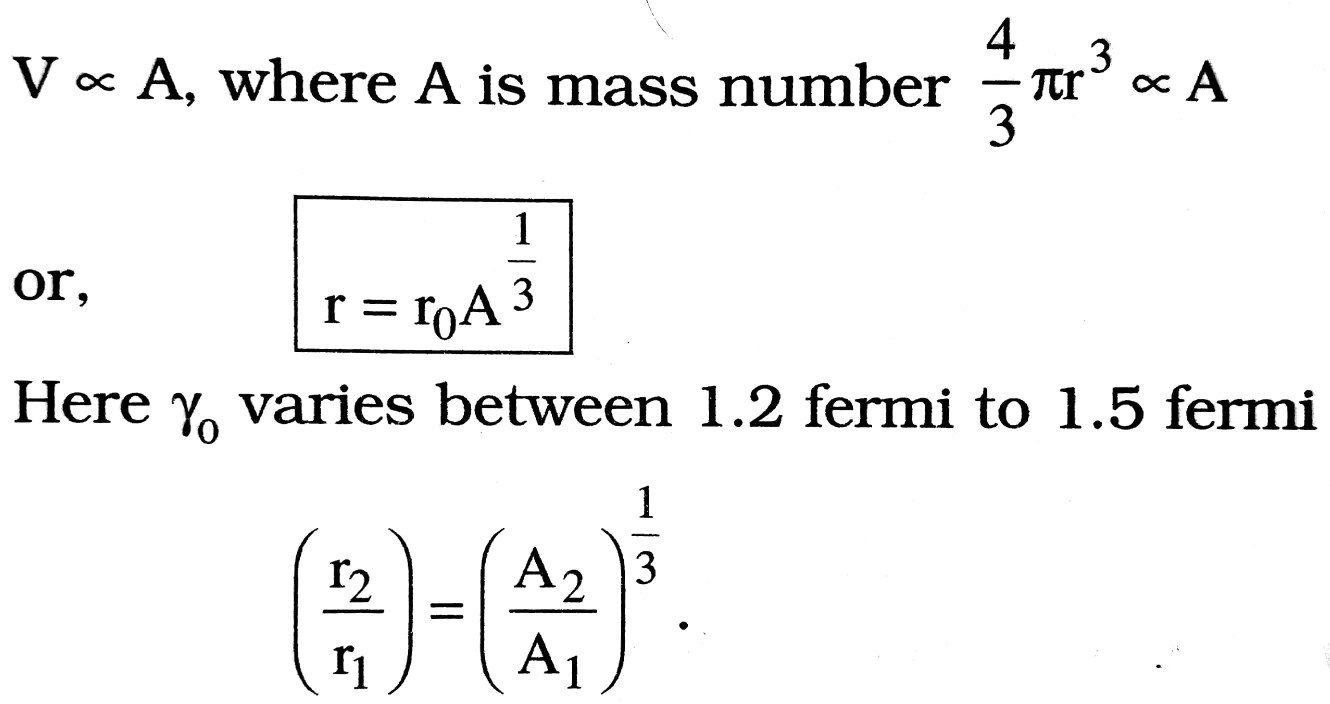The order of cubical density of a nuclues is 1017 kg/m3.

## Class 12th physics Subjective question in English

 S.N Physics Short Type Question English Medium 1. ELECTRIC CHARGES AND FIELDS 2. LECTROSTATIC POTENTIAL AND CAPACITANCE 3. CURRENT ELECTRICITY 4. MOVING CHARGES AND MAGNETISM 5. MAGNETISM AND MATTER 6. ELECTROMAGNETIC INDUCTION 7. ALTERNATING CURRENT 8. ELECTROMAGNETIC WAVES 9. RAY OPTICS AND OPTICAL INSTRUMENTS 10. WAVE OPTICS 11. DUAL NATURE OF MATTER AND RADIATION 12. ATOMS 13. NUCLEI 14. SEMI CONDUCTOR ELECTRONICS 15. COMMUNICATION SYSTEMS